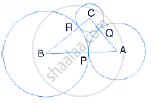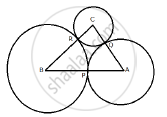Share

# Abc is a Triangle with Ab = 10 Cm, Bc = 8 Cm and Ac = 6 Cm (Not Drawn to Scale). Three Circle Are Drawn Touching Each Other with the Vertices as Their Centres. Find the Radii of the Three Circles - Mathematics

Course

#### Question

ABC is a triangle with AB = 10 cm, BC = 8 cm and AC = 6 cm (not drawn to scale). Three circle are drawn touching each other with the vertices as their centres. Find the radii of the three circles#### SolutionE
Given: ABC is a triangle with AB = 10 cm, BC= 8 cm, AC = 6 cm. Three circles are drawn with centre A, B and C touch each other at P, Q and R respectively.
We need to find the radii of the three circles.
Let
PA = AQ = x
QC = CR = y
RB = BP = z
∴ x + z = 10 ….. (1)
z + y = 8 ……..(2)
y + x = 6 …….(3)
Adding all the three equations, we have
2 (x + y + z) = 24
⇒ x + y + = 24/2 = 12        ………. (4)
Subtracting (1) (2) and (3) from (4)
y = 12 – 10 = 2
x = 12 – 8 = 4
z = 12 – 6 = 6
Therefore, radii are 2 cm, 4 cm and 6 cm

Is there an error in this question or solution?